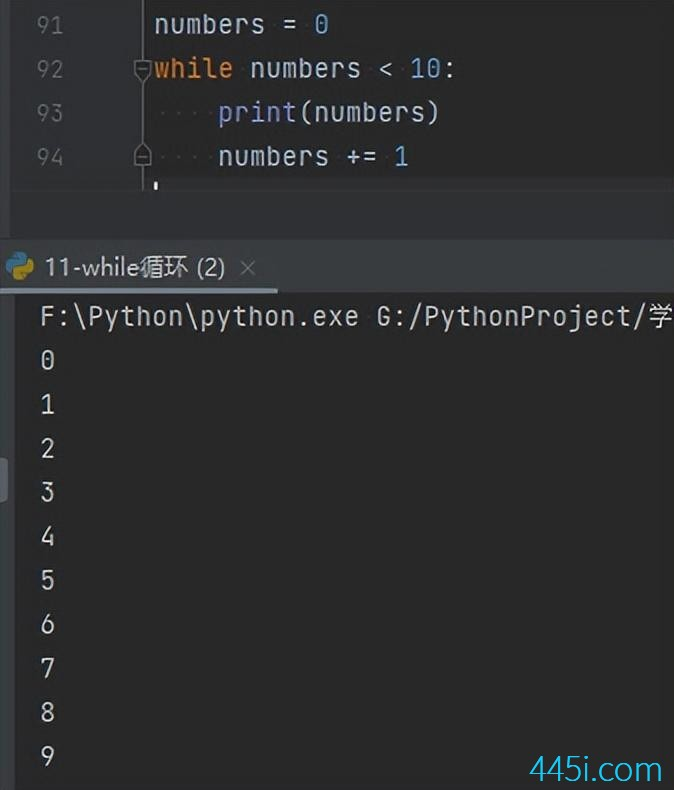# Python while与continue

continue也是结束循环的意思，不过它和break的区别是就是break是直接终止本层循环。而continue是终止本次循环，直接进入下一次循环。

``````numbers = 0
while numbers < 10:
print(numbers)
numbers += 1````````````numbers = 0
while numbers < 10:
if numbers == 5:
continue
print(numbers)
numbers += 1````````````num = 0
while num < 10:
if num == 5:
num += 1
continue
print(num)
num += 1``````### 觉得文章有用就打赏一下文章作者

#### 支付宝扫一扫打赏#### 微信扫一扫打赏